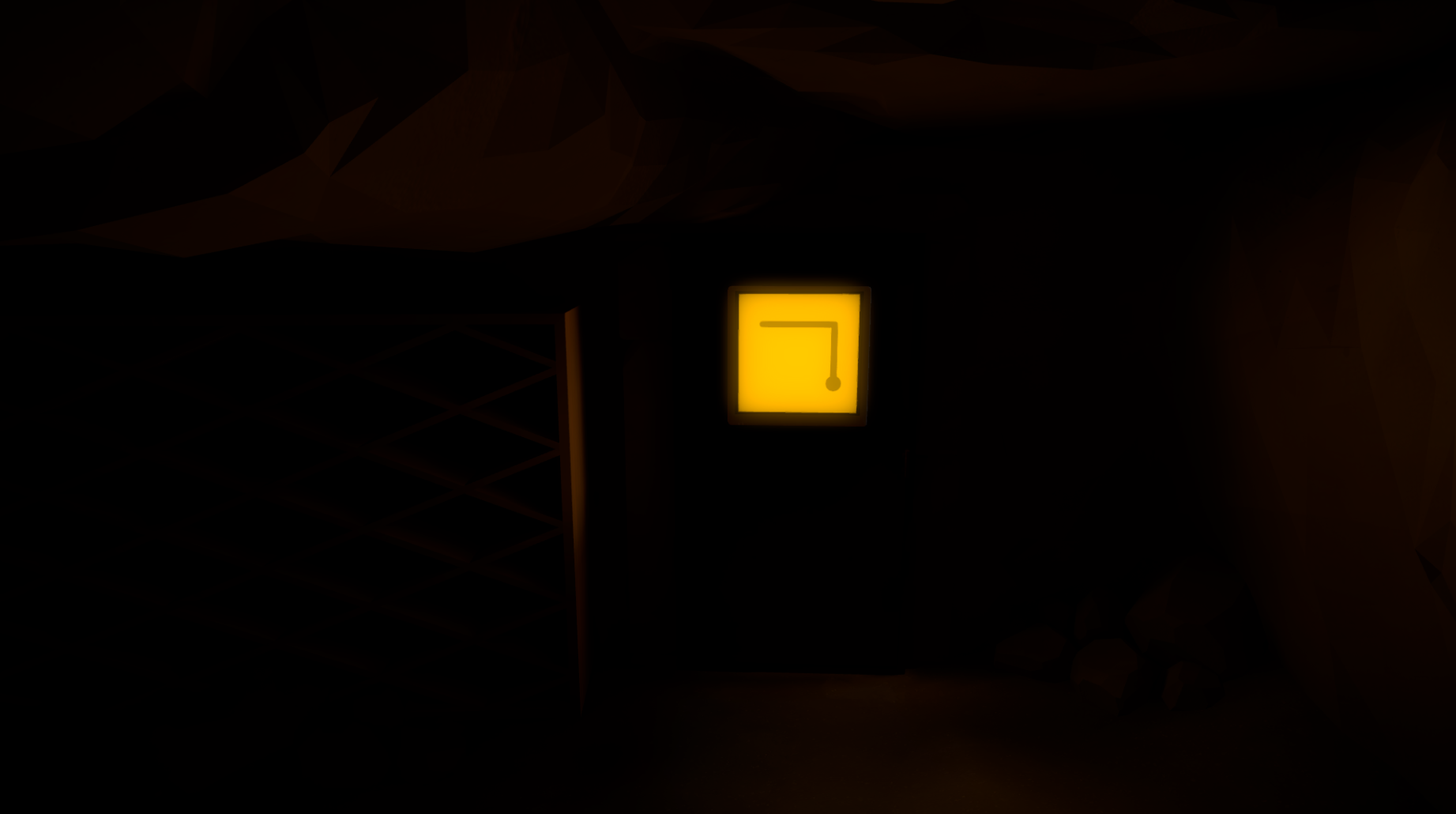## BJ & Co's Solution Guide to The  Witness

1. Staring/Entry Area

2. Orchard

3. Lake

4. Symmetry

5. Desert

6. Quarry

8. Castle/Keep

9. Shipwreck

10. Tree-house/Colored Pointy Circles

11. The Bay

12. Marsh

13. River

14. Monastery

15. Peninsula

16. Tropical Forest

17. Town/Hub

18. Mountain

###### 1. Starting Area - XXX Puzzles

The Starting Area is a nice introduction to a very simple concept. This concept is starting from the circle and finishing at a point that juts out of the puzzle with a rounded end.## Puzzle 2

### Similar to the previous puzzle all you need is to get from start to finish. nothing new introduced besides the fact that your line can make corners. Should be a quick solve if not we suggest keeping a guide handy ;)

###### 2. Orchard - XXX Puzzles

The Starting Area is a nice introduction to a very simple concept. This concept is starting from the circle and finishing at a point that juts out of the puzzle with a rounded end.

###### 3. Lake - XXX Puzzles

The Starting Area is a nice introduction to a very simple concept. This concept is starting from the circle and finishing at a point that juts out of the puzzle with a rounded end.

###### 4. Symmetry - XXX Puzzles

The Starting Area is a nice introduction to a very simple concept. This concept is starting from the circle and finishing at a point that juts out of the puzzle with a rounded end.

###### 5. Desert - XXX Puzzles

The Starting Area is a nice introduction to a very simple concept. This concept is starting from the circle and finishing at a point that juts out of the puzzle with a rounded end.

###### 6. Quarry - XXX Puzzles

The Starting Area is a nice introduction to a very simple concept. This concept is starting from the circle and finishing at a point that juts out of the puzzle with a rounded end.

###### 7. Logging/Shadows - XXX Puzzles

The Starting Area is a nice introduction to a very simple concept. This concept is starting from the circle and finishing at a point that juts out of the puzzle with a rounded end.

###### 8. Castle/Keep - XXX Puzzles

The Starting Area is a nice introduction to a very simple concept. This concept is starting from the circle and finishing at a point that juts out of the puzzle with a rounded end.

###### 9. Shipwreak - XXX Puzzles

The Starting Area is a nice introduction to a very simple concept. This concept is starting from the circle and finishing at a point that juts out of the puzzle with a rounded end.

###### 10. Treehouse/Colored Pointy Circles - XXX Puzzles

The Starting Area is a nice introduction to a very simple concept. This concept is starting from the circle and finishing at a point that juts out of the puzzle with a rounded end.

###### 11. The Bay - XXX Puzzles

The Starting Area is a nice introduction to a very simple concept. This concept is starting from the circle and finishing at a point that juts out of the puzzle with a rounded end.

###### 12. Marsh - XXX Puzzles

The Starting Area is a nice introduction to a very simple concept. This concept is starting from the circle and finishing at a point that juts out of the puzzle with a rounded end.

###### 13. River - XXX Puzzles

The Starting Area is a nice introduction to a very simple concept. This concept is starting from the circle and finishing at a point that juts out of the puzzle with a rounded end.

###### 14. Monastery - XXX Puzzles

This Site was created by the BJ and Co Team - 2016

This site was designed with the
.com
website builder. Create your website today.